# SCIENCE: Understanding Force + Motion + Gravity

THIS IS A QUIZ - IT IS NOT A TEST

It is to help you find correct answers - not to mark you down for incorrect answers!

Please think before answering each question, then skip any you don't understand.

On completing the quiz, you will receive an email (If you have supplied a
valid email address), which will include information to help you (or your
group), to find the correct solution to each incorrect or missing answer.

When you have completed the quiz, please return to the wiki at:

http://wps.flipster.org/doku.php?id=flipsters:forces:quiz:home

If you have any questions leave a wiki comment, ask classmates or a teacher.

Have fun :)

Adapted from: Science Education Assessment Resources (SEAR)

This is a required question
This is a required question
This is a required question
Must contain @
This is a required question
This is a required question

## Q1. Two EQUAL WEIGHTS are attached to each end of a rope on a PULLEY. What will happen when the boy & the girl both let go of the weights at the same time: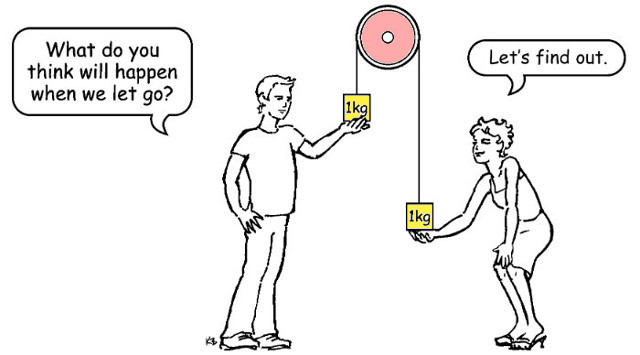This is a required question
This is a required question
 1 2 3 4 5 Confused Certain
This is a required question

## Q2. A golfer hits a ball - After the golfer has HIT the ball and ignoring any wind and air resistance, what FORCES are acting on the ball AT EACH POINT (A, B, C) as it flies through the air (along the dotted line):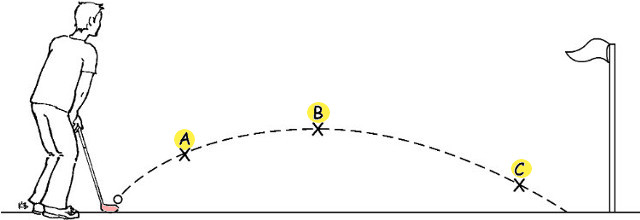This is a required question
This is a required question
This is a required question
 1 2 3 4 5 Confused Certain
This is a required question

## Q3. Sam rolled his toy car down a ramp like this: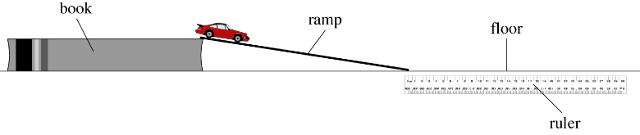## Sam changed the NUMBER OF BOOKS under the end of the ramp to change the height and angle of the ramp. The car travelled DIFFERENT DISTANCES. He measured these distances and wrote them down: The number of books and the distance rolled by the car was entered in this table:This is a required question
This is a required question
 1 2 3 4 5 Confused Certain
This is a required question

## Q4. Sam rolled two toy cars down a ramp onto a smooth, polished wooden floor: The BLUE car was TWICE AS HEAVY as the RED car. How far did the BLUE car travel?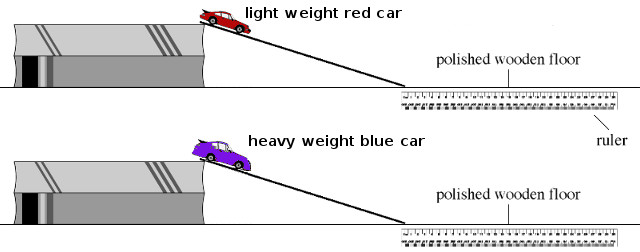This is a required question
This is a required question
 1 2 3 4 5 Confused Certain
This is a required question

## Q5. Sam noticed that when the ramp was placed on a CARPET or a POLISHED WOODEN floor the car rolled DIFFERENT DISTANCES. He measured how far the car went in each case & wrote the numbers down, but he forgot which measurement was for the carpet and which was for the polished floor. The measurements he wrote down were 31cm and 22cm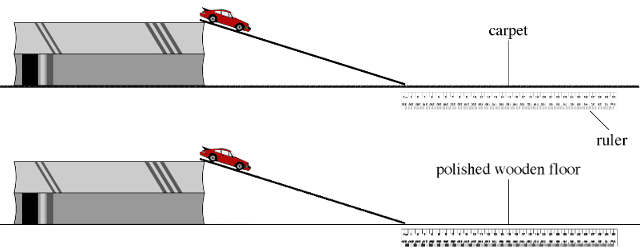This is a required question
This is a required question
This is a required question
 1 2 3 4 5 Confused Certain
This is a required question
This is a required question
 1 2 3 4 5 Confused Certain
This is a required question

## Q6. Reminder: FORCE is a push or a pull. Sam throws a ball into the air. As it travels through the air, what is the DIRECTION OF FORCE ON THE BALL as it passes point A, point B and point C (as shown on the dotted line):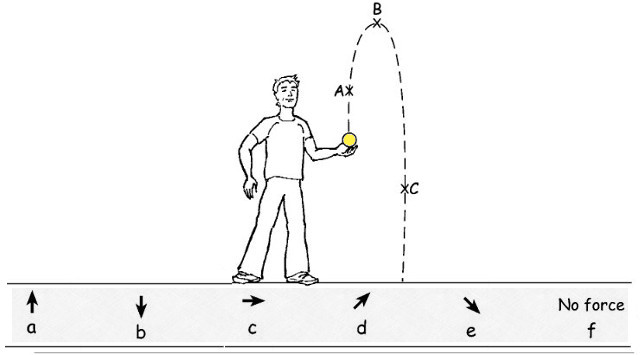This is a required question
This is a required question
This is a required question
 1 2 3 4 5 Confused Certain
This is a required question

## Q7. Two men (A, B) are pushing IDENTICAL types of cars up a hill. The velocity, wind drag and friction are IDENTICAL for each car, which man is using the MOST FORCE: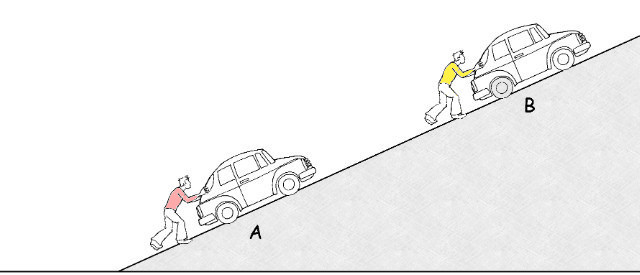This is a required question
This is a required question
 1 2 3 4 5 Confused Certain
This is a required question

## Q8. Diagrams (a, b, c, d, e, f) are how six different children drew what they thought would happen if a rock was dropped into a hole through the Earth: Ignoring heat and air pressure, which drawing SHOWS BEST what would really happen to the rock?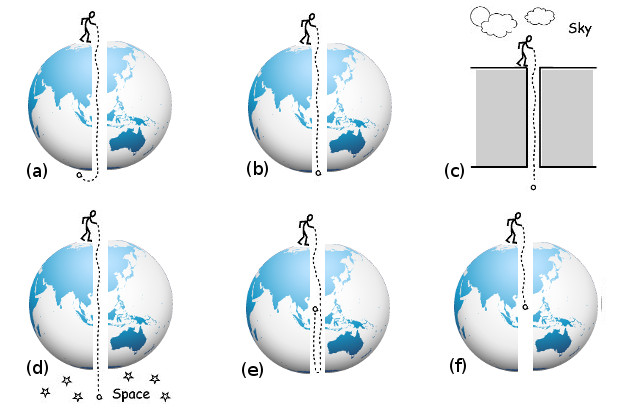This is a required question
This is a required question
 1 2 3 4 5 Confused Certain
This is a required question

## Q9.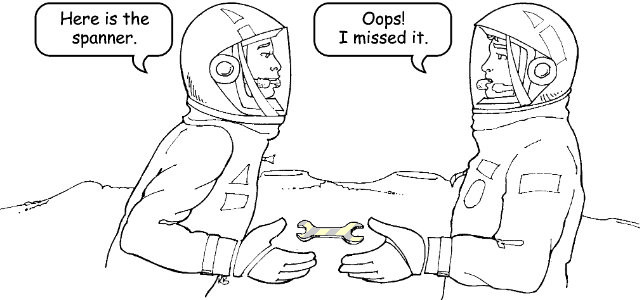This is a required question
This is a required question
 1 2 3 4 5 Confused Certain
This is a required question

## Q10. In the solar system below, there is a sun and three planets (a, b, c). The three planets are identical except they have different sizes and are different distances from the sun. An identical rocket ship is ready to leave each planet: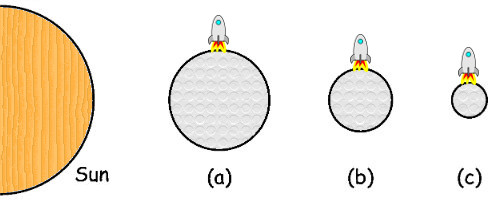This is a required question
This is a required question
 1 2 3 4 5 Confused Certain
This is a required question

## Q11. Five children drew how they thought about PEOPLE STANDING on the Earth and RAIN FALLING on the Earth. Which drawing SHOWS BEST what it is really like: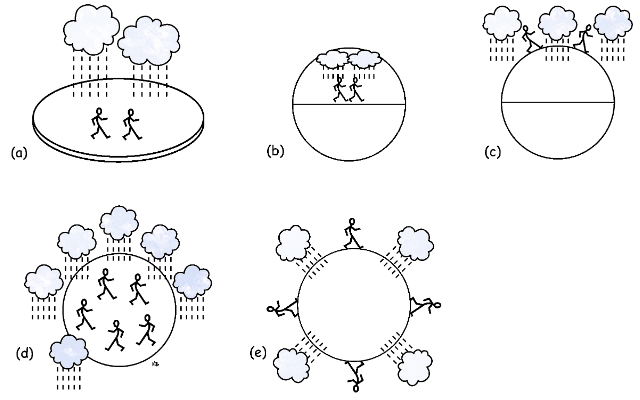This is a required question
This is a required question
 1 2 3 4 5 Confused Certain
This is a required question

## Q15. Reminder: A force is a push or a pull. A bicycle brake uses a lever and cable to cause two rubber blocks to clamp onto the side of the wheel. The bicycle rider applies a force on the brake lever to make the system work.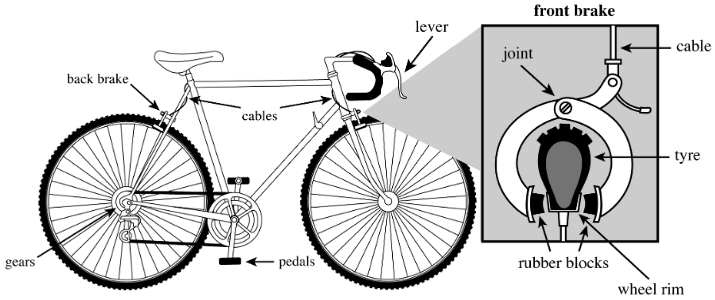This is a required question
This is a required question
This is a required question
This is a required question
This is a required question
This is a required question
 1 2 3 4 5 Confused Certain
This is a required question

## Q16. A group of students set up three separate tests for a remote-controlled toy car. For each test, the car has to travel over the same distance on three different surfaces: BITUMEN, ICE & WET GRASS. The car approaches each test surface at a fixed, steady speed. When the car reaches the start of the test surface, the students push the remote control speed lever to maximum, making the car drive wheels spin very fast.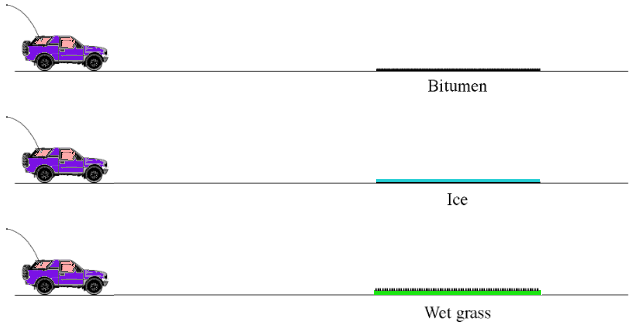This is a required question
This is a required question
This is a required question
This is a required question
 1 2 3 4 5 Confused Certain
This is a required question

## Q17. Sally moves a book across a table top at a constant, steady speed. Sally only pushes the book side-ways using her thumbs (she does not push down on the top of the book)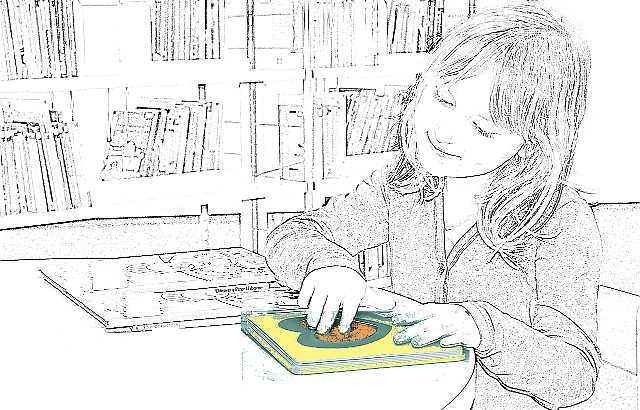This is a required question
 1 2 3 4 5 Confused Certain
This is a required question

## Q18. Boris and Doris are chicken dance partners. Looking only at the photograph, which chicken do you think has the greater mass: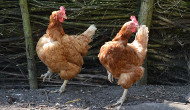This is a required question
 1 2 3 4 5 Confused Certain
This is a required question
This is a required question
This is a required question